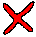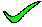The cardinality of the number of rows in the relation. The number in C ranges from the number in A times the number in B all the way to 0.

closeThe cardinality of the number of rows in the relation. The number in C ranges from the number in A times the number in B all the way to 0.

closeThe arity is the number of columns in the relation. In relational algebra, all columns of the relations are kept, and thus the arity of C is the arity of A plus B. The arity of both A and B must be greater than one.

closeThe arity is the number of columns in the relation. In relational algebra, all columns of the relations are kept, and thus the arity of C is the arity of A plus B. The arity of both A and B must be greater than one.

closeThe cardinality of the number of rows in the relation. The number in C ranges from the number in A times the number in B all the way to 0. The arity is the number of columns in the relation. In relational algebra, all columns of the relations are kept, and thus the arity of C is the arity of A plus B. The arity of both A and B must be greater than one.

close

 A B C D E TELL ME NEXT INDEX

Relation C is the join of relation A and relation B on condition p. Which of the following statements must be true in all cases?

1. The cardinality of C is greater than the cardinality of A
2. The cardinality of C is less than the cardinality of A
3. The arity of C is greater than the arity of A
4. The arity of C is less than the arity of A
5. None of the above